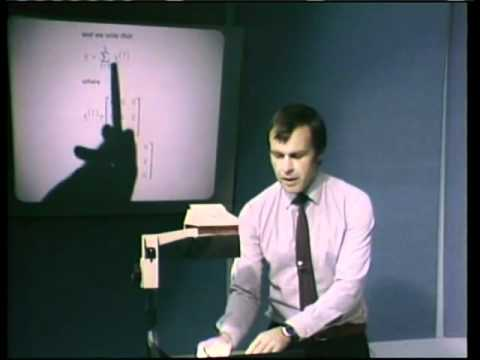MIT OpenCourseWare

# Linear Finite Element Analysis

This course introduces the basic principles used for effective finite element analysis, the general assumptions, and the implementation of finite element procedures. This video series is a comprehensive course of study that presents effective finite element procedures for the linear analysis of solids and structures. The finite element method is the ideal tool for solving static and dynamic problems in engineering and the sciences. Linear analysis assumes linear elastic behavior and infinitesimally small displacements and strains. To establish appropriate models for analysis, it is necessary to become familiar with the finite element methods available. (from ocw.mit.edu )

Instructor

###### Professor K. J. Bathe.
Mechanical engineering
2011

#### Lecture 01 - Some Basic Concepts of Engineering Analysis